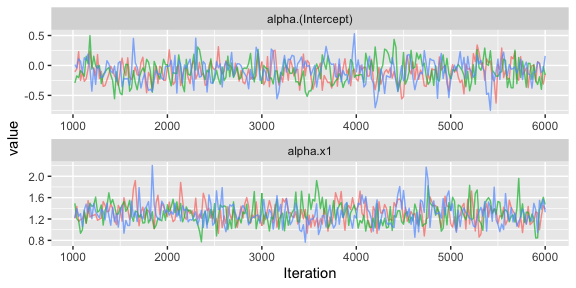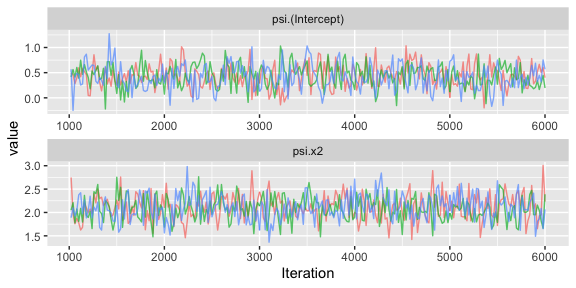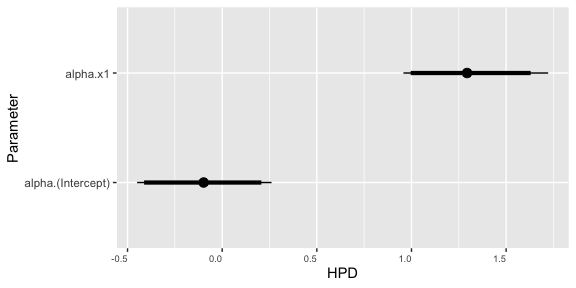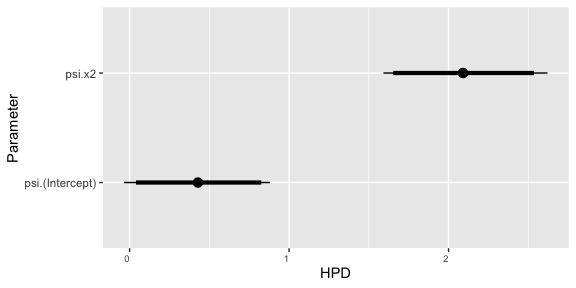# Implementation of Beta-Binomial Model

#### 2021-11-22

This package illustrates the implementation of the beta-binomial model for analyzing binomial data with overdispersion for which the level of overdisperion may vary dependent on fixed and/or random effects. As an example we consider the analysis of a simulated data set. We recommend that you work through the vignettes for the pied flycatcher data (“pied-flycatcher-1” and “pied-flycatcher-2”) which contain further information on the structure of the package.

# Beta-binomial model

The base beta-binomial model assumes that each observation, $$y_i$$, represents the number of successes from $$n_i$$ Bernoulli trials for which the probability of success, $$p_i$$, is drawn from a beta distribution. The mean and dispersion parameters for the beta distribution, $$\mu_i$$ and $$\phi_i$$, are then modelled as linear combinations of fixed and random effects.

To define the model mathematically, we must consider a non-standard parametrization of the beta distribution in terms of the mean and dispersion such that $$p \sim \mbox{Beta}(\mu,\phi)$$ implies that the expected values and variance of $$p$$ are $$\mu$$ and $$\phi \mu(1-\mu)$$. We then assume that each observation follows a binomial distribution, $$Y_i \sim \mbox{Binomial}(n_i,p_i)$$, where $$p_i \sim \mbox{Beta}(\mu_i,\phi_i)$$ such that $g(\mu_i)=\eta_i=\mathbf x_{\mu,i}^\top \mathbf \alpha + \mathbf z_{\mu,i}^\top \mathbf \epsilon$ and $h(\phi_i)=\lambda_i= \mathbf x_{\sigma,i}^\top \mathbf \psi + \mathbf z_{\sigma,i}^\top \mathbf \xi$ with $$\mathbf \alpha$$ and $$\mathbf \epsilon$$ representing vectors of fixed and random effects for the mean and $$\mathbf \psi$$ and $$\mathbf \xi$$ fixed and random effects for the dispersion. Note that both $$\mu_i$$ and $$\phi_i$$ must be between 0 and 1, and so it makes sense to assume that both $$g(\cdot)$$ and $$h(\cdot)$$ follow logistic links, or some other link function that maintains this constraint.

Given this model the marginal mean and variance of $$Y_i$$ are $$n_i\mu_i$$ and $$n_i\mu_i(1-\mu_i)[1 + (n_i-1)\phi_i]$$. In comparison to the standard binomial model (achieved by setting $$\phi_i \to 0$$ so that $$p_i=\mu_i$$) the variance is inflated by a factor of $$1 + (n_i-1)\phi_i$$.

# Library

First you have to load the package:

## Load package
library(dalmatian)

# Raw data

The raw data for this example is provided in the package and can be accessed with:

## Load beta-binomial data
data(betabin_data_1)

This data contains observations from a hypothetical study in which 50 individuals who each complete 10 replicates of some experiment consisting of 30 independent Bernoulli trials. Let $$y_{ij}$$ denote the number of successes on the $$j$$th replicate for individual $$i$$ and $$p_{ij}$$ the corresponding probability of success so that $$y_{ij} \sim \mbox{Bernoulli}(5,p_{ij})$$. The probability of success on each replicate is then assumed to follow a beta distribution with mean $$\mu_{i}=E(p_{ij})$$ defined by $\mbox{logit}(\mu_{i})=\alpha_0 + \alpha_1 x_{i1} + \epsilon_{i}$ where $$x_{i1}$$ and $$z_{i1}$$ represent individual fixed and random effects on the mean and dispersion parameter defined by $\mbox{logit}(\phi_{i})=\psi_0 + \psi_1 x_{i2} + \xi_{i}$ where $$x_{i2}$$ and $$\xi_{i}$$ represent individual fixed and random effects on the dispersion. For the simulation we set $$\alpha_0=0$$, $$\alpha_1=1$$, $$\psi_0=0$$, and $$\psi_1=2$$. We then generate the fixed and random effects as $x_{i1} \sim \mbox{Normal}(0,1)$ $x_{i2} \sim \mbox{Normal}(0,1)$ $\epsilon_{i} \sim \mbox{Normal}(0,1)$ $\xi_{i} \sim \mbox{Normal}(0,1).$

# Defining the Model

To illustrate the beta-binomial we fit the data generating model to the simulated data. The model structure is defined through two lists specifying the fixed and random effects for the mean and dispersion components:

## Define mean and variance objects
mymean <- list(fixed = list(name = "alpha",
formula = ~x1,
priors = list(c("dnorm",0,.001))),
random = list(name = "epsilon",
formula = ~ID - 1),

mydisp <- list(fixed = list(name = "psi",
formula = ~x2,
priors = list(c("dnorm",0,.001))),
random = list(name = "xi",
formula = ~ID - 1),
link = "logit")

# Running the Model with dalmatian

Once the model structure has been defined the model can be fit with the function dalmatian. The following code creates the lists of arguments supplied to jags.model and coda.samples and then calls dalmatian to run the MCMC sampler. Note that the beta-binomial model is fit by specifying that family="betabin". For the beta-binomial model it is necessary to specify the name of the variable in the data set that contains the number of trials for each observation (in this case “m”). Though the number of trials is constant in the simulated data this is not necessary.

## Set working directory
## By default uses a system temp directory. You probably want to change this.
workingDir <- tempdir()

## Define list of arguments for jags.model()
jm.args <- list(file=file.path(workingDir,"betabin_test_1.R"),n.chains = 3, n.adapt = 1000)

## Define list of arguments for coda.samples()
cs.args <- list(n.iter=5000,thin=20)

## Run the model using dalmatian
bbmcmc <- dalmatian(df=betabin_data_1,
family = "betabin",
mean.model=mymean,
dispersion.model=mydisp,
jags.model.args=jm.args,
coda.samples.args=cs.args,
response = "y",
ntrials = "m",
residuals = FALSE,
run.model = TRUE,
engine = "JAGS",
n.cores = 3,
overwrite = TRUE,
saveJAGSinput = workingDir)
## Registered S3 method overwritten by 'GGally':
##   method from
##   +.gg   ggplot2

# Results

Once the samples have been generated the post-processing functions can be used to examine the behaviour of the sampler and compute posterior summary statistics. These functions are described further in the help pages and in the vignettes analyzing the pied flycatcher data.

## Convergence Diagnostics

## Compute convergence diagnostics
bbconvergence <- convergence(bbmcmc)
## Gelman-Rubin diagnostics
bbconvergence$gelman ## Potential scale reduction factors: ## ## Point est. Upper C.I. ## alpha.(Intercept) 1.01 1.01 ## alpha.x1 1.00 1.00 ## psi.(Intercept) 1.00 1.01 ## psi.x2 1.00 1.01 ## sd.epsilon.ID 1.00 1.02 ## sd.xi.ID 1.00 1.01 ## Raftery diagnostics bbconvergence$raftery
##
## Quantile (q) = 0.025
## Accuracy (r) = +/- 0.005
## Probability (s) = 0.95
##
## You need a sample size of at least 3746 with these values of q, r and s
## Effective sample size
bbconvergence$effectiveSize ## alpha.(Intercept) alpha.x1 psi.(Intercept) psi.x2 ## 454.1882 532.9845 553.7584 751.0542 ## sd.epsilon.ID sd.xi.ID ## 522.4797 750.0000 ## Traceplots ## Generate traceplots ## bbtraceplots <- traceplots(bbmcmc,show=FALSE) ## Fixed effects for mean bbtraceplots$meanFixed## Fixed effects for dispersion
bbtraceplots$dispersionFixed## Numerical Summaries ## Compute numerical summaries bbsummary <- summary(bbmcmc) ## Print numerical summaries bbsummary ## ## Iterations = 1020:6000 ## Thinning interval = 20 ## Number of chains = 3 ## Sample size per chain = 250 ## ## Posterior Summary Statistics for Each Model Component ## ## Mean Model: Fixed Effects ## Mean Median SD Lower 95% Lower 50% Upper 50% Upper 95% ## (Intercept) -0.1 -0.10 0.19 -0.45 -0.25 -0.02 0.26 ## x1 1.3 1.29 0.20 0.93 1.15 1.40 1.69 ## ## Mean Model: Random Effects ## Mean Median SD Lower 95% Lower 50% Upper 50% Upper 95% ## ID 1.12 1.11 0.16 0.83 0.97 1.17 1.47 ## ## Dispersion Model: Fixed Effects ## Mean Median SD Lower 95% Lower 50% Upper 50% Upper 95% ## (Intercept) 0.43 0.43 0.24 -0.03 0.25 0.56 0.89 ## x2 2.10 2.09 0.27 1.61 1.88 2.25 2.63 ## ## Dispersion Model: Random Effects ## Mean Median SD Lower 95% Lower 50% Upper 50% Upper 95% ## ID 1.34 1.32 0.23 0.96 1.13 1.41 1.83 ## Graphical Summaries ## Generate caterpillar bbcaterpillar <- caterpillar(bbmcmc,show = FALSE) ## Fixed effects for mean bbcaterpillar$meanFixed## Fixed effects for dispersion
bbcaterpillar\$dispersionFixed